Monotonous functions are integrable

Which bounded functions are integrable?

This is a difficult question to answer. One simple partial answer is that monotonic functions are integrable.

A function is monotonic on an interval if it is either increasing or decreasing on the interval. If f is a monotonic function on [a,b] then it is bounded and integrable. (And the result can be easily generalized to bounded piecewise monotonic functions [see Ross, p. 196]).

"Fortunately, most of the functions that occur in practice are monotonic or sums of monotonic functions, so the result of this miniature theory of integration is quite comprehensive." (Apostol, p. 76)

In the applet you can play with a simple case of monotonic functions: continuous monotonic functions, but the result is true in general. (By the way, another important family of integrable functions are all continuous functions on [a,b]).

In the applet it is possible to modify the curve and the ends of integration, to increase or to diminish the number of rectangles, to show the error we make and a bound of that error. We can see an animation about that.

Before considering the theoretical aspect of the problem we can think a little about the approximation of the value of the definite integral of a monotonic function.

When approximating an integral using rectangles we make an error. In some cases, for example, in monotonic functions, we can limit the magnitude of the error. The error is equal to the sum of the areas of the blue "curved triangles".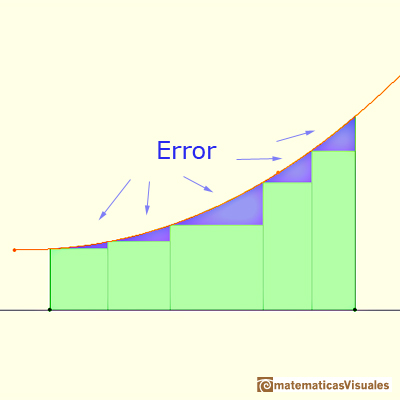This error is less than the area of a rectangle.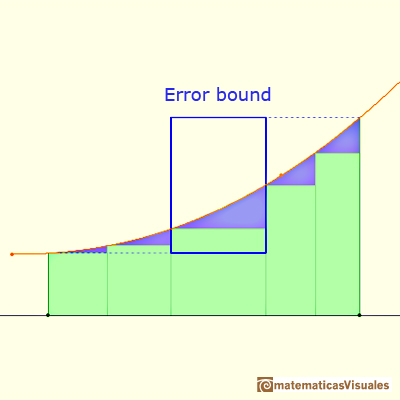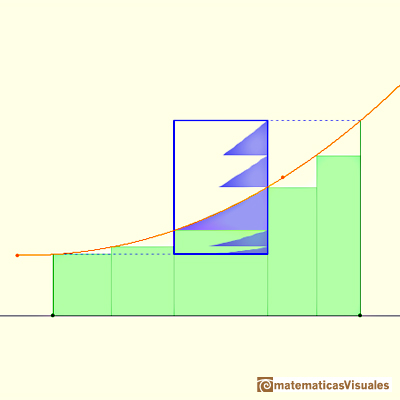If we consider a simple case, when the bases of the rectangles are equal, then we can calculate an error bound:Using refined partitions we obtain as small bounds of the error as we want. Because when we refine a partition the base of the rectangle is smaller.Now we are going to prove that a monotonic positive increasing function on [a,b] is integrable.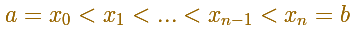If we divide the interval into n equal parts then the width of each rectangle is:

We can calculate an upper bound of the integral: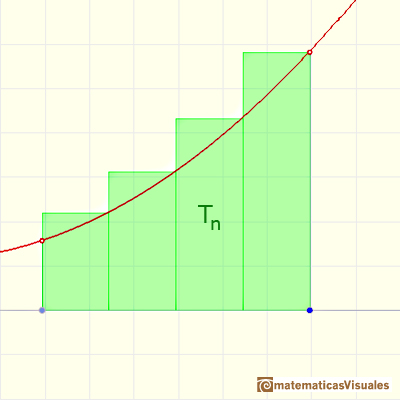We consider the decreasing sequence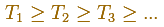With the same partition, this is a lower bound of the integral:We consider the increasing sequenceIf this two sequences converge toward one and the same limit, we can call this limit the definite integral, and writeBut the question always is whether they do converge.

When we consider an increasing monotonic function we have an increasing sequence (Sn) and a decreasing sequence (Tn). Then the question is only whether we can verifyWe can calculate Tn - Sn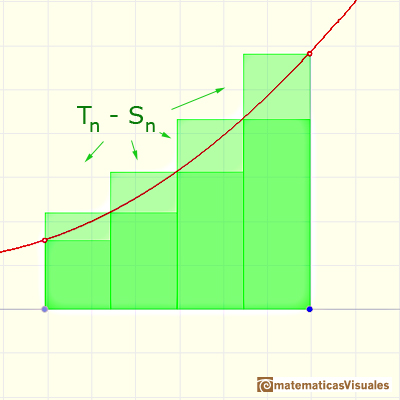Then the limit

We conclude that if f is monotonic in the interval [a,b], then the definite integral exists. [Toeplitz, p.64]This is Newton's approach to the question in 'The Mathematical Principles of Natural Philosophy' ('Principia'). You can read this book in Google books (p. 17), California Digital Library or a latin version in Cambridge Digital Library, for example.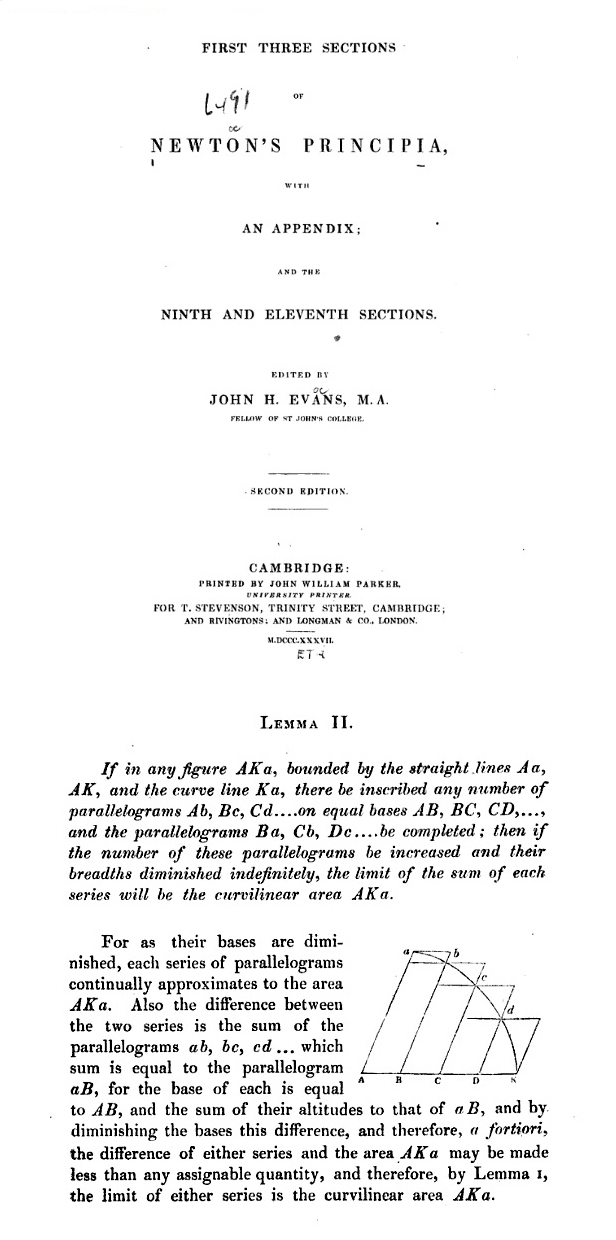REFERENCES

Markushevich, Áreas y logaritmos. Ed. Mir.
Tom M. Apostol, Calculus, Second Edition, John Willey and Sons, Inc. (p. 77).
Michael Spivak, Calculus, Third Edition, Publish-or-Perish, Inc. (p. 256)
Otto Toeplitz, The Calculus, a genetic approach, The University of Chicago Press, 1963 (p. 63).
Kenneth A. Ross, Elementary Analysis: The Theory of Calculus, Springer-Verlag New York Inc., 1980 (p. 190).If we consider the lower limit of integration a as fixed and if we can calculate the integral for different values of the upper limit of integration b then we can define a new function: an indefinite integral of f.It is easy to calculate the area under a straight line. This is the first example of integration that allows us to understand the idea and to introduce several basic concepts: integral as area, limits of integration, positive and negative areas.To calculate the area under a parabola is more difficult than to calculate the area under a linear function. We show how to approximate this area using rectangles and that the integral function of a polynomial of degree 2 is a polynomial of degree 3.We can see some basic concepts about integration applied to a general polynomial function. Integral functions of polynomial functions are polynomial functions with one degree more than the original function.The Fundamental Theorem of Calculus tell us that every continuous function has an antiderivative and shows how to construct one using the integral.The Second Fundamental Theorem of Calculus is a powerful tool for evaluating definite integral (if we know an antiderivative of the function).As an introduction to Piecewise Linear Functions we study linear functions restricted to an open interval: their graphs are like segments.A piecewise function is a function that is defined by several subfunctions. If each piece is a constant function then the piecewise function is called Piecewise constant function or Step function.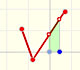A continuous piecewise linear function is defined by several segments or rays connected, without jumps between them.If the derivative of F(x) is f(x), then we say that an indefinite integral of f(x) with respect to x is F(x). We also say that F is an antiderivative or a primitive function of f.Archimedes show us in 'The Method' how to use the lever law to discover the area of a parabolic segment.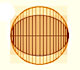In his book 'On Conoids and Spheroids', Archimedes calculated the area of an ellipse. We can see an intuitive approach to Archimedes' ideas.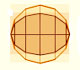In his book 'On Conoids and Spheroids', Archimedes calculated the area of an ellipse. It si a good example of a rigorous proof using a double reductio ad absurdum.Kepler used an intuitive infinitesimal approach to calculate the area of a circle.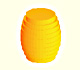Kepler was one mathematician who contributed to the origin of integral calculus. He used infinitesimal techniques for calculating areas and volumes.Studying the volume of a barrel, Kepler solved a problem about maxima in 1615.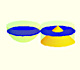Using Cavalieri's Principle we can calculate the volume of a sphere.Howard Eves's tetrahedron is Cavalieri congruent with a given sphere. You can see that corresponding sections have the same area. Then the volumen of the sphere is the same as the volume of the tetrahedron. And we know how to calculate this volumen.